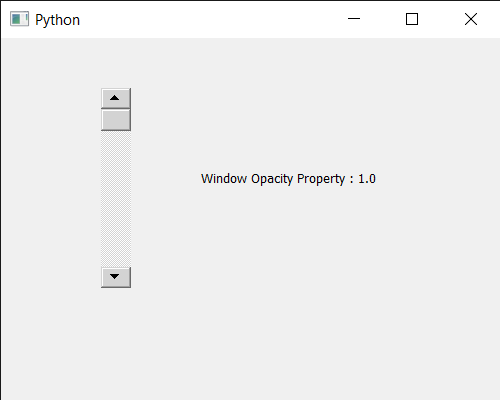GFG App
Open AppBrowser
Continue

# PyQt5 QScrollBar – Getting Window Opacity property

In this article we will see how we can get the window opacity property of the QScrollBar. QScrollBar is a control that enables the user to access parts of a document that is larger than the widget used to display it. Slider is the scroll-able object inside the bar. This property holds the level of opacity for the window. The valid range of opacity is from 1.0 (completely opaque) to 0.0 (completely transparent). It can be set with the help of setWindowOpacity method.

In order to do this we will use windowOpacity method with the scroll bar object.

Syntax : scroll.windowOpacity()

Argument : It takes no argument

Return : It returns float value

Below is the implementation

## Python3

 `# importing libraries` `from` `PyQt5.QtWidgets ``import` `*` `from` `PyQt5 ``import` `QtCore, QtGui` `from` `PyQt5.QtGui ``import` `*` `from` `PyQt5.QtCore ``import` `*` `import` `sys`     `class` `Window(QMainWindow):`   `    ``def` `__init__(``self``):` `        ``super``().__init__()`   `        ``# setting title` `        ``self``.setWindowTitle("Python ")`   `        ``# setting geometry` `        ``self``.setGeometry(``100``, ``100``, ``500``, ``400``)`   `        ``# calling method` `        ``self``.UiComponents()`   `        ``# showing all the widgets` `        ``self``.show()`       `    ``# method for components` `    ``def` `UiComponents(``self``):`   `        ``scroll ``=` `QScrollBar(``self``)`   `        ``# setting geometry of the scroll bar` `        ``scroll.setGeometry(``100``, ``50``, ``30``, ``200``)`   `        ``# making its background color to green` `        ``scroll.setStyleSheet("background : lightgrey;")`   `        ``# setting window opacity property` `        ``scroll.setWindowOpacity(``1.0``)`   `        ``# creating a label` `        ``label ``=` `QLabel("GeeksforGeeks", ``self``)`   `        ``# setting geometry to the label` `        ``label.setGeometry(``200``, ``100``, ``300``, ``80``)`   `        ``# making label multi line` `        ``label.setWordWrap(``True``)`   `        ``# getting value changed signal` `        ``scroll.valueChanged.connect(``lambda``: do_action())`   `        ``# method called when signal is emitted` `        ``def` `do_action():` `            `  `            ``# setting text to the label` `            ``label.setText("Current Value : " ``+` `str``(scroll.value()))`   `        ``# getting window opacity property` `        ``value ``=` `scroll.windowOpacity()`   `        ``# setting text to the label` `        ``label.setText("Window Opacity ``Property` `: " ``+` `str``(value))`     `# create pyqt5 app` `App ``=` `QApplication(sys.argv)`   `# create the instance of our Window` `window ``=` `Window()`   `# start the app` `sys.exit(App.``exec``())`

Output :My Personal Notes arrow_drop_up